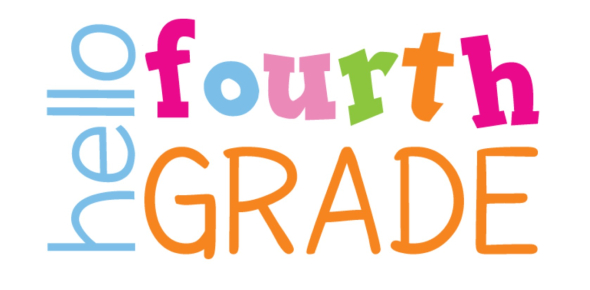# 4th Grade IQ Test Quiz

Approved & Edited by ProProfs Editorial Team
The editorial team at ProProfs Quizzes consists of a select group of subject experts, trivia writers, and quiz masters who have authored over 10,000 quizzes taken by more than 100 million users. This team includes our in-house seasoned quiz moderators and subject matter experts. Our editorial experts, spread across the world, are rigorously trained using our comprehensive guidelines to ensure that you receive the highest quality quizzes.
| By Grace Paszkiewicz
G
Grace Paszkiewicz
Community Contributor
Quizzes Created: 1 | Total Attempts: 38,163
Questions: 9 | Attempts: 38,993SettingsDo you think you'll be able to solve this 4th grade IQ test? If yes, then you must check out the quiz below. The fourth grade might sound like it would be easy to go through as an adult, but the children of today can easily have a rough time going through it considering the number of topics been thrown at them. If you want to help your kid study, then this quiz should help you do the trick! Just answer the questions, and we’ll do the rest!

• 1.

• A.

17

• B.

18

• C.

20

A. 17
Explanation
The correct answer is 17 because 12+5 equals 17 in basic arithmetic.

Rate this question:

• 2.

### If you have two cookies and four people, what fraction would be equal to everyone? (Write in fraction)

1/2
Explanation
If there are 2 cookies and 4 people, each person can only have a fraction of a cookie. To determine the fraction that would be equal to everyone, we divide the total number of cookies (2) by the total number of people (4). This gives us 1/2 or one half. Therefore, each person would get 1/2 of a cookie, which would be equal for everyone.

Rate this question:

• 3.

### State true or false. 3x3=9

• A.

True

• B.

False

A. True
Explanation
The statement "3x3=9" is true because when you multiply 3 by itself, the result is indeed 9.

Rate this question:

• 4.

### John has three boxes of cookies to share with his family of four. Each box has three cookies. If you were to divide that between the family how much would each get equally?

• A.

2.25

• B.

3.35

• C.

4.45

A. 2.25
Explanation
Each box has 3 cookies, so in total, John has 3 x 3 = 9 cookies. He needs to share these cookies equally among his family of 4. To divide equally, he would give each person 9 / 4 = 2.25 cookies.

Rate this question:

• 5.

### Which planet is known as the "Red Planet" in our solar system?

• A.

Venus

• B.

Mars

• C.

Jupiter

• D.

Saturn

B. Mars
Explanation
Mars is the correct answer. It is known as the "Red Planet" because of its reddish appearance, caused by iron oxide (rust) on its surface.

Rate this question:

• 6.

### State whether true or false. 4X12= 36

• A.

True

• B.

False

B. False
Explanation
The statement "4X12= 36" is false because the product of 4 multiplied by 12 is actually equal to 48, not 36.

Rate this question:

• 7.

### Abc_efghijklmnop_rstuvwxyz Which two letters are missing?

• A.

Dv

• B.

Dq

B. Dq
Explanation
The given string is a sequence of letters from a to z, with the letters "d" and "q" missing. This can be deduced by comparing the given string with the alphabet sequence and identifying the missing letters. Therefore, the correct answer is "dq".

Rate this question:

• 8.

### Fill in the blank with the correct article. ______ Taj Mahal is located in India.

• A.

The

• B.

An

• C.

A

• D.

Their

A. The
Explanation
The correct answer is "The". The Taj Mahal refers to a specific monument, and the definite article "The" is used to indicate that it is a unique and well-known landmark.

Rate this question:

• 9.

### Fill in the blank with the appropriate pronoun here.  Naira sent a bouquet of flowers to _____ mother.

• A.

His

• B.

Her

• C.

Myself

• D.

Their

B. Her
Explanation
The correct answer is "Her" because Naira sent the bouquet of flowers to someone else's mother, indicating that the person's mother is a female. Therefore, the appropriate pronoun to use in this context is "Her."

Rate this question:

Related TopicsBack to top